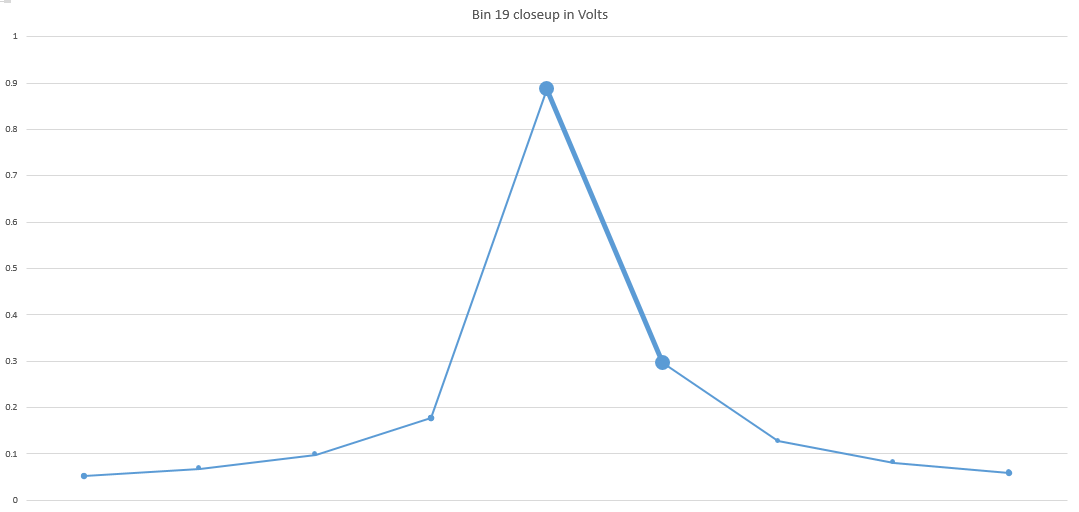Non-coherent fundamental

# Fun With Bunnemann

Published on October 19, 2019

At the end of a previous article I suggested that rather than windowing, oscilloscope makers try something more akin to what we do in the ATE biz by trying to approximate coherent sampling. When you sample coherently, you don't get the smearing that comes with non-coherent sampling, like this, with an M of 19.25;This plot shows the spectrum of a wave that has been clipped on the positive peak, and if you have ever read any of my articles here, you may recognize it as this one captured coherently: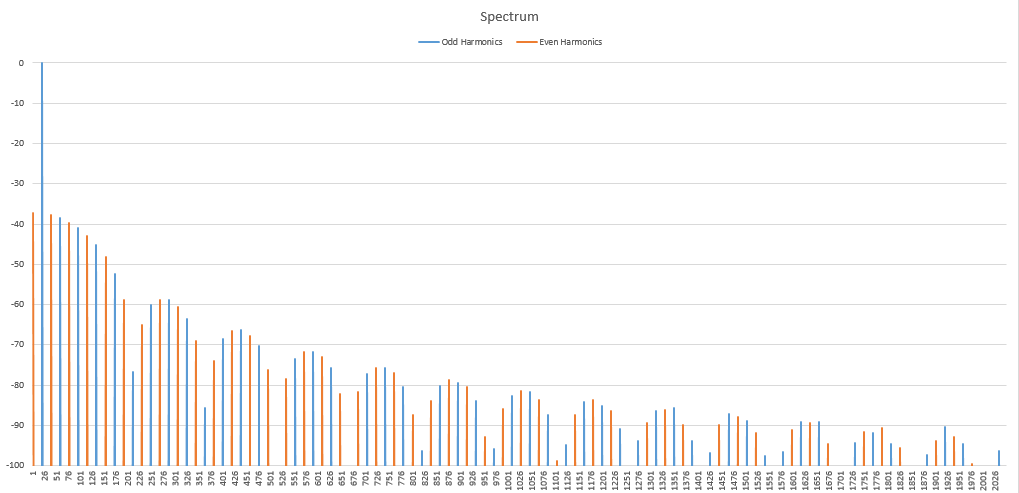The two don't look very much alike, but I assure you that the time domain wave looks identical, and the distortion is identical. Also the lower one is color coded blue for Odd harmonics, red for Even harmonics, but that's pretty hard to do in the upper one, because the wave was captured non-coherently, so the energy is spread out among several bins.

Now because the non-coherent spectrum is so useless, most spectrum analyzer and oscilloscope makers apply a window to the wave before performing the FFT. Here I apply a Blackman-Harris window and then do the FFT, and you can see that my result looks a lot more like the coherent version: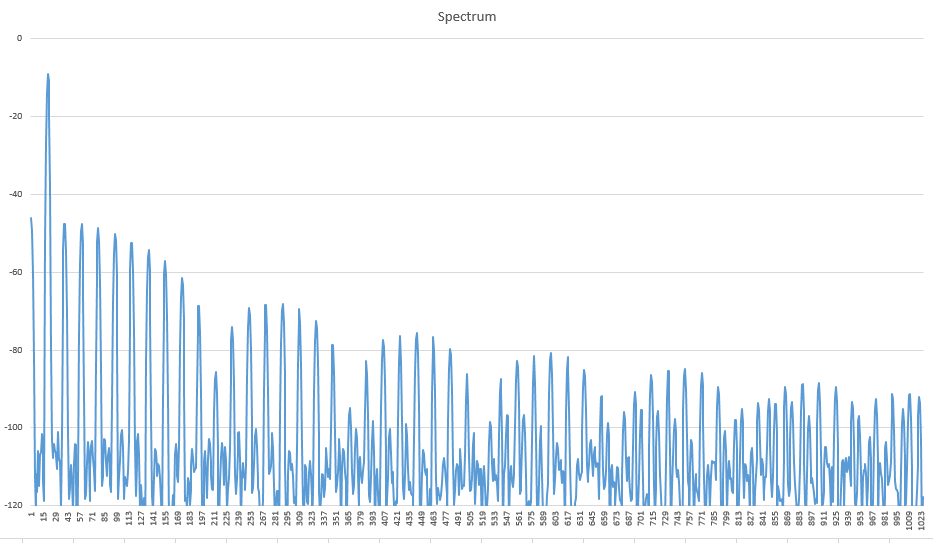Now, one thing to note right away, this spectrum and the non-coherent spectrum are missing something else that my nice clean coherent spectrum has: That one has unfolded the aliases that you seen running across the bottom of this plot. Count the number of humps here: Six and a half, right? Count the number of humps in the coherent spectrum: Thirteen, right? So in both of the non-color coded spectrums, I didn't bother unwinding the first order aliases, so the spectrums appear half as wide as the pretty, coherent and color coded spectrum, but otherwise they are the same. Aliasing can be used to your advantage, it's nothing to be afraid of, as long as you know Dan's Rules.

While the windowed spectrum is nicer, it's still not very useful, especially if you want to discover the true cause of harmonic distortion. It's OK for scope dopes, people who believe that an FFT is just a button on a scope, but for real engineers and scientists, windowing, while useful is kind of a crutch. But how can we get around this problem? It's pretty hard to coherently sample a wave when you don't control the clock base rate of the signal you are investigating, like on a 'scope. Ah, but there is a trick that I talk about in my article on measuring duty cycle without a Time Measurement Unit. It's a trick called Bunnemann that I learned from David Reynolds, discoverer of Daver's Law. Here is how it works.We capture non-coherently, without windowing, do the FFT, convert to voltage (not dB), and find the fundamental which is generally the largest signal (but not always, be careful!). Then we look at the two bins on each side of the fundamental. We are looking for the two biggest signals out of the three, and in this case, the biggest one is the fundamental in bin 19, and the second biggest is the signal in bin 20. What that tells us is that the number of cycles of the wave was 19.xxx, but we don't know what that xxx is. But Bunnemann can help us find out. Here is what I did in Excel to work out the number of cycles using Bunnemann.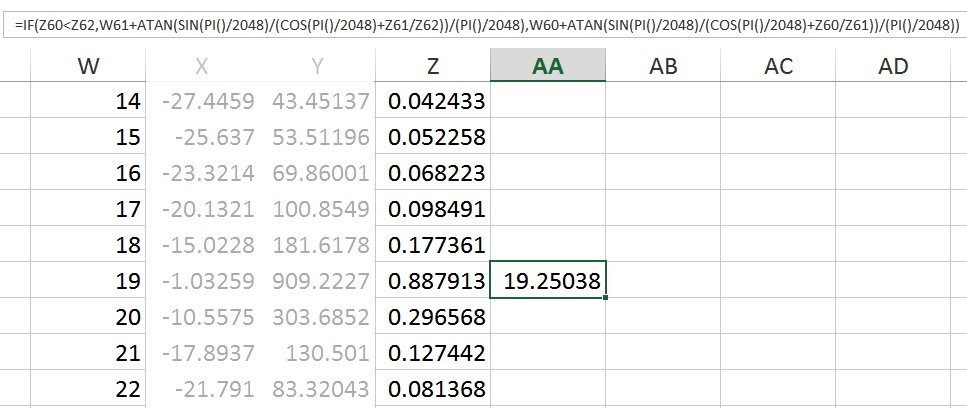In column W is the bin number, in column Z is the voltage from my spectrum (do this in volts, not dB) and in column AA I have calculated the number of cycles that I captured with Bunnemann. The Bunnemann formula looks like this:

### bin_num = left_bin+atan(sin(pi/N)/(cos(pi/N)+left_mag/right_mag))/(pi/N);

Where N is the number of samples captured. Now the left_bin and right_bin vary depending on which two of the three (center and the skirts) are bigger. In this case, the upper bin, bin 20 (aka Z62) is larger than bin 18 (aka Z60) meaning that we captured 19.xxx cycles of the wave, not 18.xxx cycles of the wave. That means that left_bin is 19. If bin 18 had been larger than bin 20, we would have used bin 18 for the left_bin and the largest signal, the fundamental would be the right_bin.

So Bunnemann tells us that our number of cycles is 19.25038, which is off a bit from the actual value of 19.25. The reason it's off is this: remember all those aliases? They (along with noise in a real-world environment) change the values ever so slightly and that changes the true value of the real number of cycles. So, A)How can we use this information and B)What can we do about the inaccuracy of our measurement.

To answer question A, we can adjust our sampling frequency so that we capture an integer number of cycles. If you want to be really lazy, just sample 4 times slower, that means that that we will end up with 77 cycles of the wave and magically the fundamental will show up in bin 77. In fact, remember that this was a distorted wave, which has harmonics. Take a look at a tight version of the non-windowed spectrum;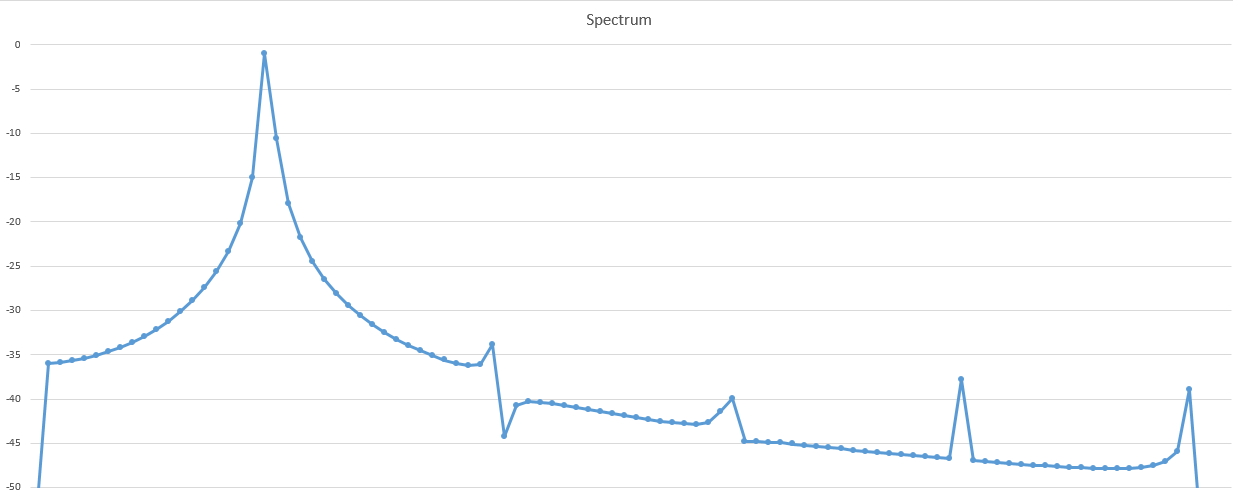Here you can see the fundamental on the far left, then the second harmonic, then the third, and the fourth and finally the fifth. But notice the 4th harmonic; if you had to try to apply Bunnemann to that one it would be tough to choose which was going to be the right and the left bin because the skirts looks pretty even. Why? Because the 4th harmonic should fall in 4*19.25 or bin 77. And the exact same thing that would happen to the fundamental if we slowed the sampling down by a factor of 4. Let me just quickly mention that we don't apply Bunnemann to harmonics because we need lots of amplitude to get as close as we can, and harmonics are by definition smaller than the fundamental (except in rare cases as the article I mentioned above explains).

There are other ways to change the sampling rate. And realize that there are other ways to attain an integer number of cycles. One way is to do the math thusly;

### 19.25/2048 = 19/2021.402597

But can you do an FFT on 2021.402597 samples? No, but you can do it on 2021 samples using a Chirp-Z transform which takes any number of samples, even an odd number. So you take the wave you have now, no resampling needed, truncate it to 2021 samples, do a Chirp-Z on it and look at the spectrum again. Because we truncated our N by 0.402587 samples, the real number of cycles is 18.99622, really, really close to exactly 19. In other words we were off by a quarter of a cycle before, now we are off by 3.78 thousandths of a cycle, a huge improvement. So how did it work out?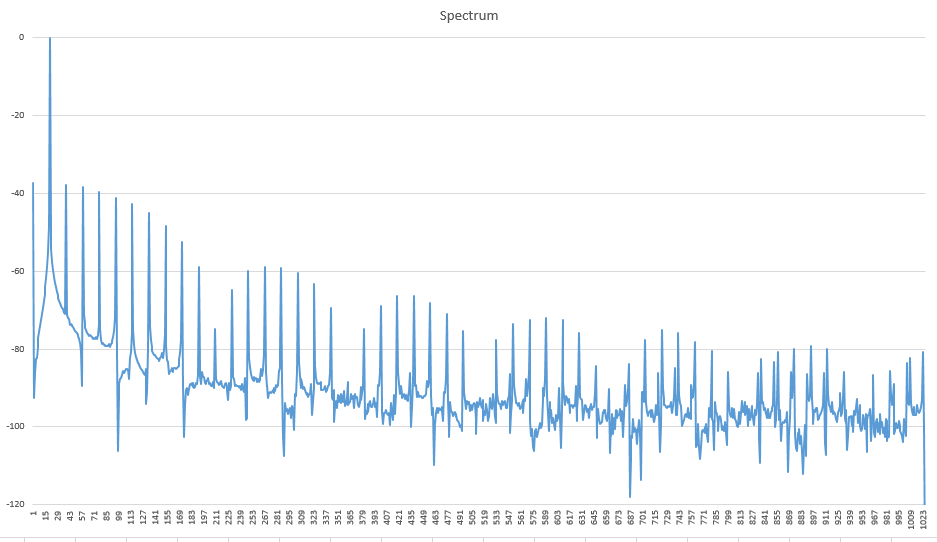Wow, that's pretty good! Better than our windowed version, virtually no spreading at all! Compare it to the Windowed 19.25 cycle spectrum:See, the Blackman-Harris window causes the each harmonic in the spectrum to spread a bit, not a whole lot, but far more than if you optimize the M (the number of cycles) to a near-integer value. Now, can the un-windowed spectrum be color coded like the real coherent version?Yes, it works! It does really well up until after we start aliasing, then it falls apart because of the early spreading at the front of the spectrum. But hey, if you don't like the way it looks, do Bunnemann again and figure out what to do from there. Change sampling frequency, recalculate a new number of samples, do a new Chirp-Z transform, and see how it looks. You don't have to be perfectly coherent, but closer is better. And if you want to window, go ahead, just don't use it in every single case. It seriously compromises the spectrum. It's OK for looking at Signal to Noise measurements, but it's not really good for most other things because it seriously impacts the amplitude of the signal, and I thought that is what you were looking at! This is one reason that nobody ever figured out how harmonics work, they were looking at the output of a windowed spectrum and there are enough inaccuracies that add up to make your results unrepeatable and not tied to any reality. See, there is an advantage to using simulations and knowing the difference between simulations and real world measurements.